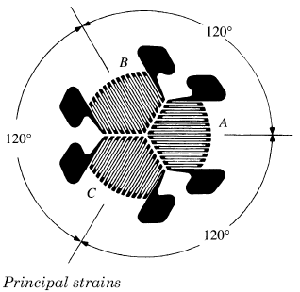Related Resources: calculators

### Three-Element Delta Rosette Strain Gage Equations and Calculator

Three-Element Delta Rosette Strain Gage Equations and Calculator

The principal strains and stresses are given relative to the xy coordinate axes as shown below.Three-Element Rectangular Rosette

Maximum Principal StrainMinimum Principal StrainPrincipal Stresses:

Maximum Principal StressMinimum Principal StressTreating the tan-1 as a single-valued function, the angle counterclockwise from gage A to the axis containing εp1 or σp1 is given by:Where:

E = Young's Modulus (N/m2, lbs/in2)
ν = Poissons Ratio
ε = strain (μm/m, μin/in) µ
σ = Stress (N/m2, lbs/in2)
θp = Angle (degrees)

Reference:

Roarks Formulas for Stress and Strain
KYOWA Electronic Instruments